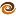EmptyCrate

About Me | Contact Me | Training |ChaiScript.com | YouTube Channel

In C++, the virtual keyword, when applied to class methods, aids in polymorphism. If a method is declared to be virtual, the most derived version of the method is executed when a call is made. If a method is non-virtual, the specific version that is called depends on how the method is called. If the method is called via a pointer to the base class, the base class method is called, if by the derived class, then the derived method is called. This definition is weak; an example is better, as usual:

#include <iostream>
#include <string>

struct Base {
// Non virtual method
int getInt()   { return 1; }

// Virtual Method
virtual std::string getString()   { return "Base"; }
};

struct Derived : Base {
int getInt()   { return 2; }

virtual std::string getString()   { return "Derived"; }
};

int main() {
Derived d;
Base &baseref(d);
Base *baseptr(&d);
Derived &derivedref(d);
Derived *derivedptr(&d);

// Calling by the object itself, the expected version is called
std::cout << d.getInt() << std::endl; // 2
std::cout << d.getString() << std::endl;  // "Derived"

// Calling by a reference or pointer to the base class,
// non-virtual methods call the base class version
// virtual methods call the derived version
std::cout << baseref.getInt() << std::endl; // 1
std::cout << baseref.getString() << std::endl;  // "Derived"
std::cout << baseptr->getInt() << std::endl;  // 1
std::cout << baseptr->getString() << std::endl;  // "Derived"

// Calling by a reference or pointer to the derived class
// The derived versions are always called
std::cout << derivedref.getInt() << std::endl;  // 2
std::cout << derivedref.getString() << std::endl;  // "Derived"
std::cout << derivedptr->getInt() << std::endl; // 2
std::cout << derivedptr->getString() << std::endl;  // "Derived"
}

Taking the above classes:

Base *b = new Derived();
delete b; // Warning, non-virtual destructor

If we were to delete a Derived by a pointer to Base, only the Base destructor is called! This means the Derived destructor is not called and the object is not properly freed. Most modern compilers will warn if you create this situation. The fix to it is simple. You most simply provide virtual destructors:

struct Base
{
// We have virtual methods, so we should provide a virtual destructor
virtual ~Base()
{
}
// Non virtual method
int getInt()
{
return 1;
}

// Virtual Method
virtual std::string getString()
{
return "Base";
}
};

struct Derived : Base
{
virtual ~Derived()
{
}

int getInt()
{
return 2;
}

virtual std::string getString()
{
return "Derived";
}
};
My Videos